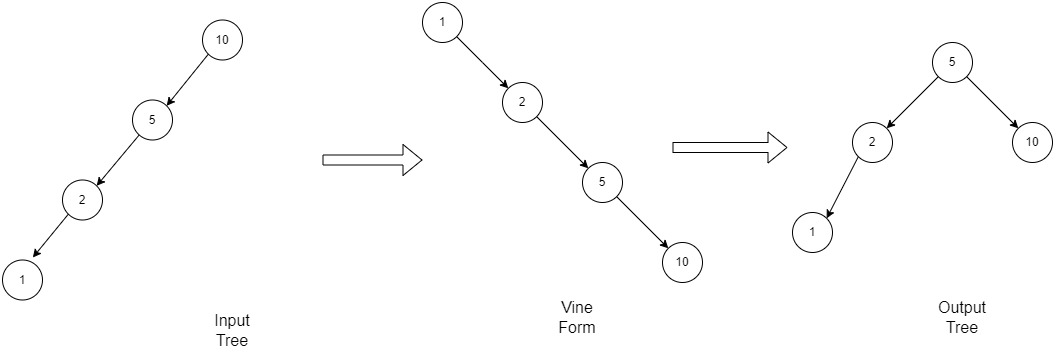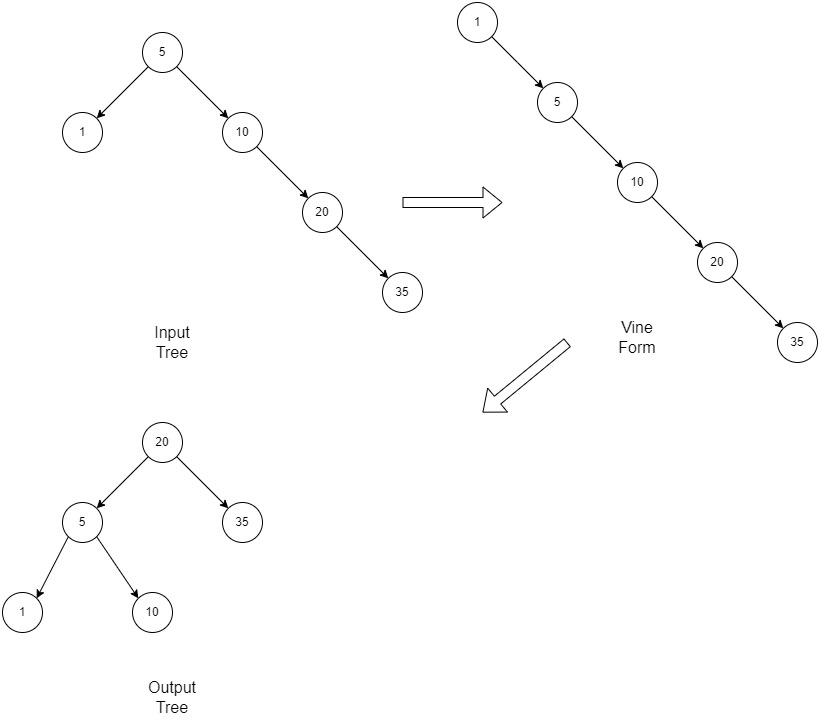# Day-Stout-Warren algorithm to balance given Binary Search Tree

• Difficulty Level : Medium
• Last Updated : 19 Jan, 2022

Given an unbalanced Binary Search Tree (BST), the task is to convert it into a balanced BST in linear time and without using auxiliary space.

Examples:

Input:               5
/  \
1   10
\
20
\
35
Output:          20
/   \
5     35
/   \
1      10

Input:                 10
/
5
/
2
/
1
Output:            5
/  \
2    10
/
1

Approach: The algorithm used in this scenario is the Day-Stout-Warren algorithm. The balanced tree formed will be a complete binary tree. Follow the steps below for the implementation of the algorithm.

• Step 1: Convert the given BST into a linked list ( right-sided linked list ) using the concept of right rotations by means of inorder traversal. This form of BST is known as backbone or vine. the Runtime of this phase is linear and no extra space is required.
The function is coded in such a way that it does all the required right rotation to flatten the BST and at the end returns the number of nodes in BST.
• Step 2: Calculate the height of BST in which all the levels will be completely filled using the formula h = log2(N+1) [N is the total number of nodes]. And using the height calculate the number of nodes that can be fitted in that height m = pow(2, h)-1. [h is height till which all the levels are fully filled with nodes]
The difference (diff) of N and m is the amount of nodes that will be there in last level of balanced complete binary tree.
• The vine obtained in the first step is then left rotated diff amount of time from its root. The above modified tree is then left rotated m/2, m/4, m/8 . . . times until m is greater than 0 according to the algorithm

Illustrations:

Illustration-1: Here the given tree is a left skewed BSTExample-1

Illustration-2: Here it is a non-skewed but unbalanced BSTExample-2

Below is the implementation of the above approach.

## C++

 `// C++ code to balance BST using DSW algorithm.``#include ``using` `namespace` `std;`` ` `// Defining the structure for TreeNode.``struct` `TreeNode {``    ``int` `val;``    ``TreeNode* left;``    ``TreeNode* right;``    ``TreeNode()``        ``: val(0)``        ``, left(NULL)``        ``, right(NULL)``    ``{``    ``}``    ``TreeNode(``int` `x)``        ``: val(x)``        ``, left(NULL)``        ``, right(NULL)``    ``{``    ``}``    ``TreeNode(``int` `x, TreeNode* left, ``             ``TreeNode* right)``        ``: val(x)``        ``, left(left)``        ``, right(right)``    ``{``    ``}``};`` ` `// Function to convert input BST ``// to right linked list``// known as vine or backbone.``int` `bstToVine(TreeNode* grand)``{``    ``int` `count = 0;`` ` `    ``// Make tmp pointer to traverse ``    ``// and right flatten the given BST.``    ``TreeNode* tmp = grand->right;`` ` `    ``// Traverse until tmp becomes NULL``    ``while` `(tmp) {``         ` `        ``// If left exist for node ``        ``// pointed by tmp then ``        ``// right rotate it.``        ``if` `(tmp->left) {``            ``TreeNode* oldTmp = tmp;``            ``tmp = tmp->left;``            ``oldTmp->left = tmp->right;``            ``tmp->right = oldTmp;``            ``grand->right = tmp;``        ``}`` ` `        ``// If left dont exists``        ``// add 1 to count and ``        ``// traverse further right to``        ``// flatten remaining BST.``        ``else` `{``            ``count++;``            ``grand = tmp;``            ``tmp = tmp->right;``        ``}``    ``}`` ` `    ``return` `count;``}`` ` `// Function to compress given tree``// with its root as grand->right.``void` `compress(TreeNode* grand, ``int` `m)``{``    ``// Make tmp pointer to traverse ``    ``// and compress the given BST.``    ``TreeNode* tmp = grand->right;`` ` `    ``// Traverse and left-rotate root m times ``    ``// to compress given vine form of BST.``    ``for` `(``int` `i = 0; i < m; i++) {``        ``TreeNode* oldTmp = tmp;``        ``tmp = tmp->right;``        ``grand->right = tmp;``        ``oldTmp->right = tmp->left;``        ``tmp->left = oldTmp;``        ``grand = tmp;``        ``tmp = tmp->right;``    ``}``}`` ` `// Function to implement the algorithm``TreeNode* balanceBST(TreeNode* root)``{``    ``// create dummy node with value 0``    ``TreeNode* grand = ``new` `TreeNode(0);`` ` `    ``// assign the right of dummy node as our input BST``    ``grand->right = root;`` ` `    ``// get the number of nodes in input BST and``    ``// simultaneously convert it into right linked list.``    ``int` `count = bstToVine(grand);`` ` `    ``// gets the height of tree in which all levels``    ``// are completely filled.``    ``int` `h = log2(count + 1);`` ` `    ``// get number of nodes until second last level``    ``int` `m = ``pow``(2, h) - 1;`` ` `    ``// Left rotate for excess nodes at last level``    ``compress(grand, count - m);`` ` `    ``// Left rotation till m becomes 0``    ``// Step is done as mentioned in algo to``    ``// make BST balanced.``    ``for` `(m = m / 2; m > 0; m /= 2) {``        ``compress(grand, m);``    ``}`` ` `    ``// return the balanced tree``    ``return` `grand->right;``}`` ` `// Function to print preorder traversal``// of Binary tree.``void` `preorderTraversal(TreeNode* root)``{``    ``if` `(!root)``        ``return``; ``    ``cout << root->val << ``" "``;``    ``preorderTraversal(root->left); ``    ``preorderTraversal(root->right);``    ``return``;``}`` ` `// Driver code``int` `main()``{``    ``TreeNode* root = ``new` `TreeNode(10);``    ``root->left = ``new` `TreeNode(5);``    ``root->left->left = ``new` `TreeNode(2);``    ``root->left->left->left ``        ``= ``new` `TreeNode(1);``     ` `    ``// Function call to implement ``    ``// Day-Stout-Warren algorithm``    ``root = balanceBST(root);``     ` `    ``// To print the preorder traversal of BST``    ``preorderTraversal(root);`` ` `    ``return` `0;``}`

## Javascript

 ``

Output
`5 2 1 10 `

Time Complexity: O(N) where N is the total number of nodes in the BST
Auxiliary Space: O(1)

My Personal Notes arrow_drop_up